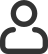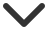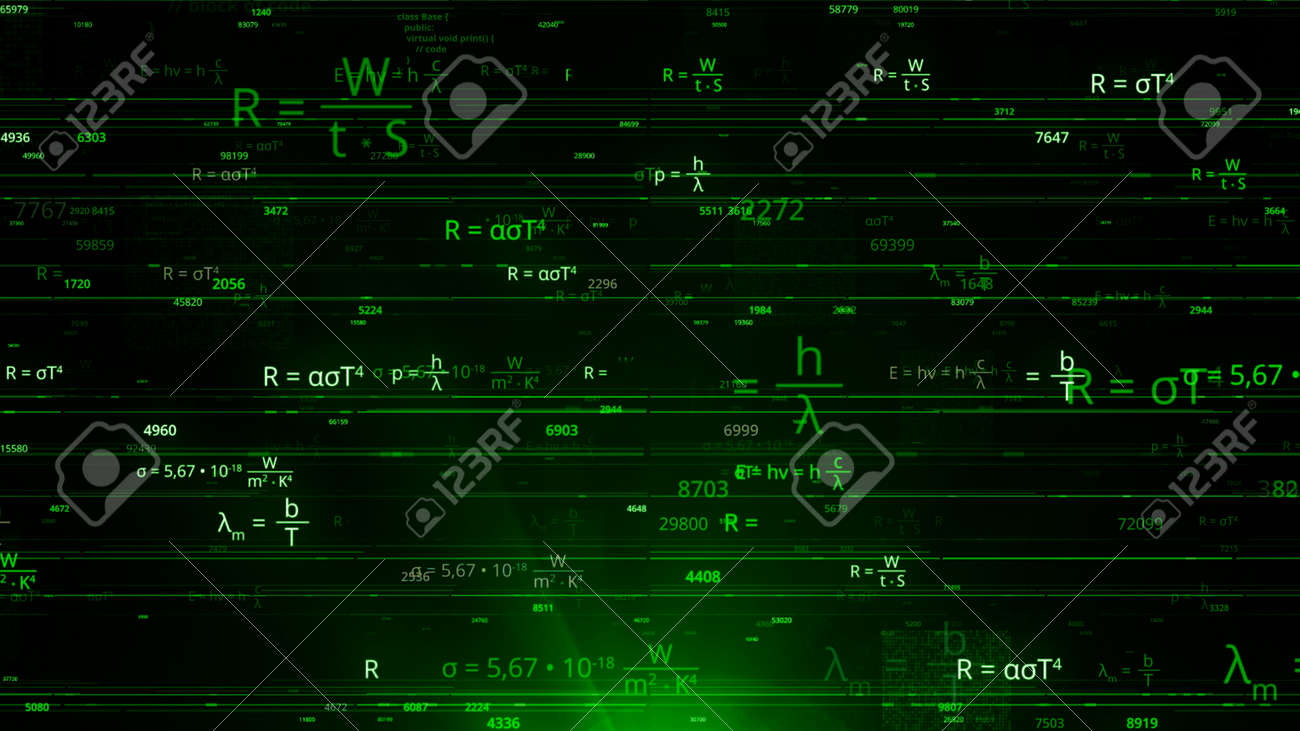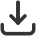Merhaba, Guest
Diller
Türkçe# Stok Fotoğraf — Many of mathematical formulas in matrix. Animation. Matrix network with changing mathematical equations. Cybernetic field with mathematical equationsBoyut
Standart Boyutlar
S923 x 519 px • 72 dpi923 x 519 px12.82 x 7.21 inches
M2731 x 1536 px • 300 dpi2731 x 1536 px9.1 x 5.12 inches
L3840 x 2160 px • 300 dpi3840 x 2160 px12.8 x 7.2 inches
XL5760 x 3240 px • 300 dpi5760 x 3240 px19.2 x 10.8 inches
Görüntü bilgisi## Many of mathematical formulas in matrix. Animation. Matrix network with changing mathematical equations. Cybernetic field with mathematical equations

Görsel Kimliği: 169163118
Medya Türü: Stok Fotoğraf
Telif hakkı: davidoff205020